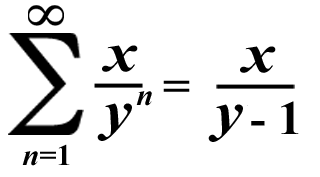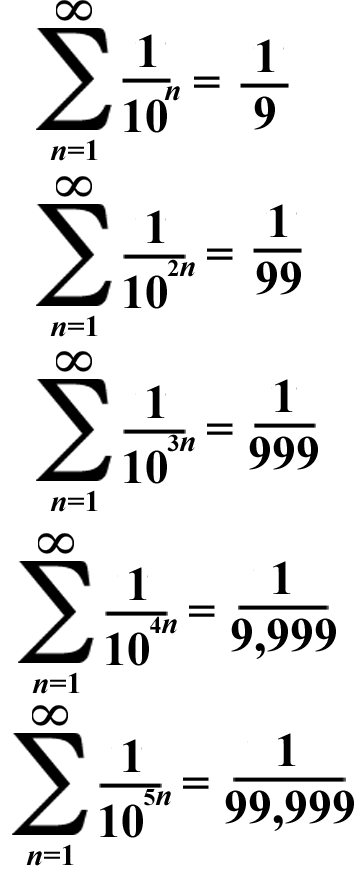# Powerful Patterns 3: Summation with the Negative Powers of 10

## This math trick references the 1st Super Summation trick! Here's the summation formula from that math trick again:### Let x = 1 & y = 10 this time. Also, we're going to multiply the variable n by consecutive integers! (That are greater than zero(0) of course)## The common ratio of each summation above is a negative power of 10. Each consecutive negative power of 10 adds another 9 to the denominator of the fraction!